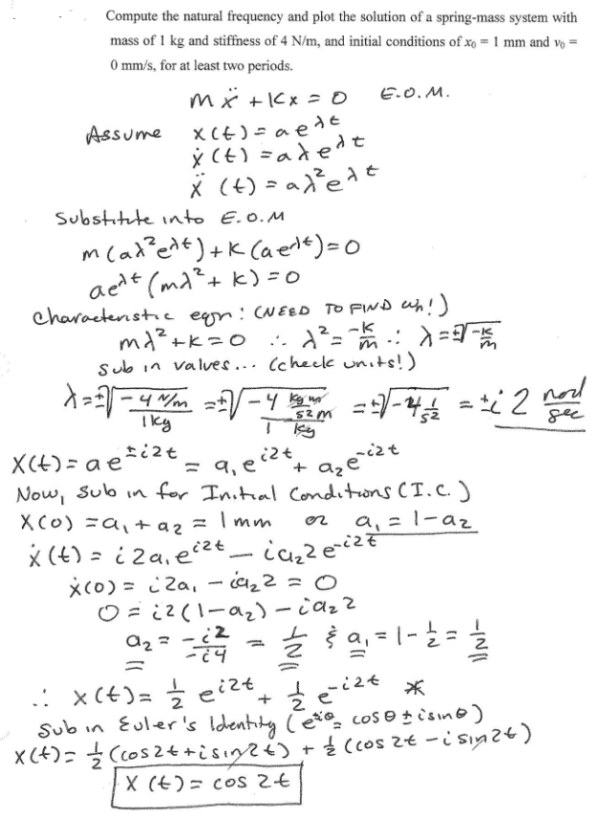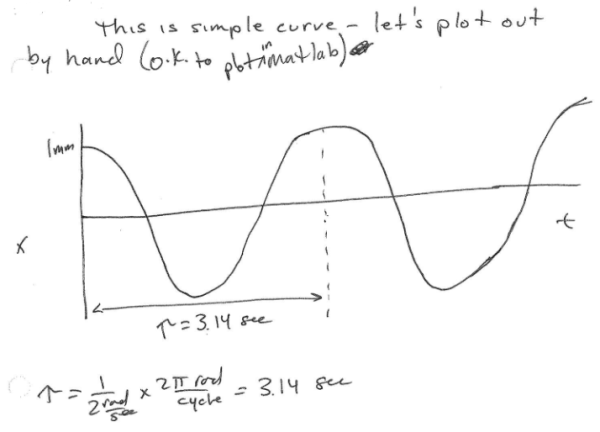Compute the natural frequency and plot the soution of a sping-mass system with mass of 1 kg and stiffness of 4 N/m, and initial conditions of x0=1mm and v0=0 mm/s, for at least two periods.Compute the natural frequency and plot the soution of a sping-mass system with mass of 1 kg and stiffness of 4 N/m, and initial conditions of x0=1mm and v0=0 mm/s, for at least two periods.

Vibrations Page 1 vibrations vibrations vibrations vibrations vibrations vibrations vibrations Vibrations Page 2